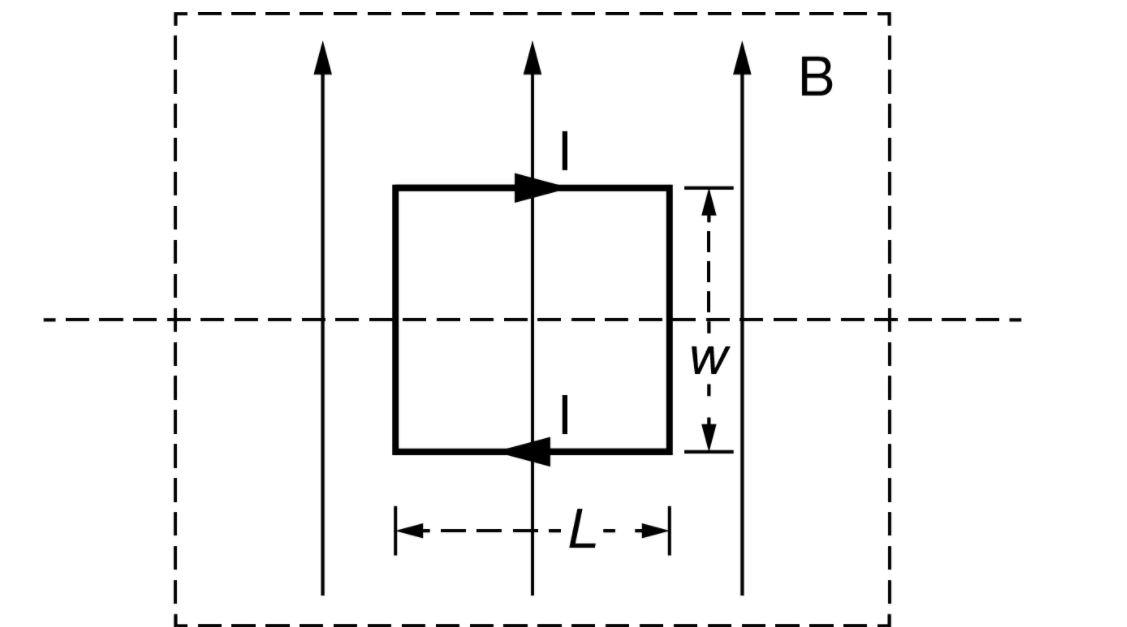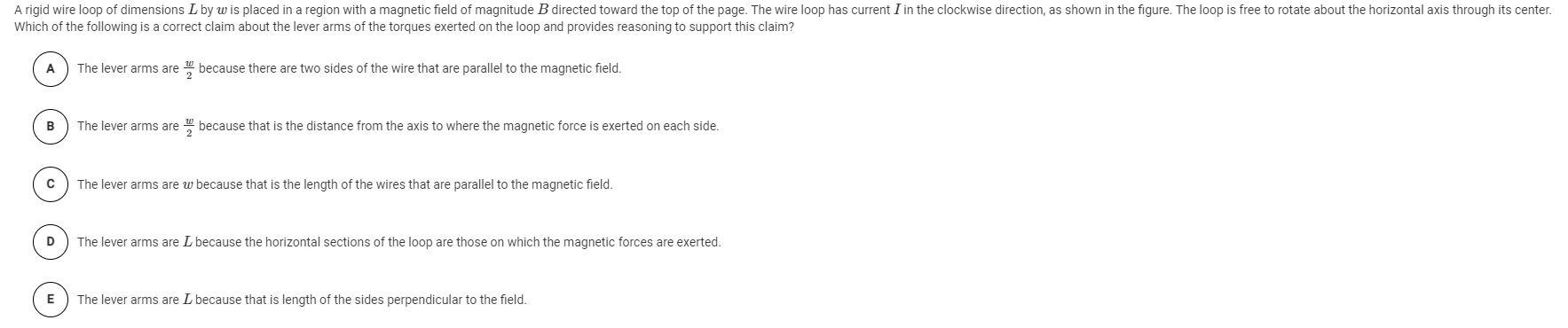# Question Solved1 AnswerB 1 M I I |--- |--7- A rigid wire loop of dimensions L by w is placed in a region with a magnetic field of magnitude B directed toward the top of the page. The wire loop has current I in the clockwise direction, as shown in the figure. The loop is free to rotate about the horizontal axis through its center. Which of the following is a correct claim about the lever arms of the torques exerted on the loop and provides reasoning to support this claim? A The lever arms are because there are two sides of the wire that are parallel to the magnetic field. B The lever arms are because that is the distance from the axis to where the magnetic force is exerted on each side. The lever arms are w because that is the length of the wires that are parallel to the magnetic field. D The lever arms are L because the horizontal sections of the loop are those on which the magnetic forces are exerted. E The lever arms are L because that is length of the sides perpendicular to the field.Transcribed Image Text: B 1 M I I |--- |--7- A rigid wire loop of dimensions L by w is placed in a region with a magnetic field of magnitude B directed toward the top of the page. The wire loop has current I in the clockwise direction, as shown in the figure. The loop is free to rotate about the horizontal axis through its center. Which of the following is a correct claim about the lever arms of the torques exerted on the loop and provides reasoning to support this claim? A The lever arms are because there are two sides of the wire that are parallel to the magnetic field. B The lever arms are because that is the distance from the axis to where the magnetic force is exerted on each side. The lever arms are w because that is the length of the wires that are parallel to the magnetic field. D The lever arms are L because the horizontal sections of the loop are those on which the magnetic forces are exerted. E The lever arms are L because that is length of the sides perpendicular to the field.
More
Transcribed Image Text: B 1 M I I |--- |--7- A rigid wire loop of dimensions L by w is placed in a region with a magnetic field of magnitude B directed toward the top of the page. The wire loop has current I in the clockwise direction, as shown in the figure. The loop is free to rotate about the horizontal axis through its center. Which of the following is a correct claim about the lever arms of the torques exerted on the loop and provides reasoning to support this claim? A The lever arms are because there are two sides of the wire that are parallel to the magnetic field. B The lever arms are because that is the distance from the axis to where the magnetic force is exerted on each side. The lever arms are w because that is the length of the wires that are parallel to the magnetic field. D The lever arms are L because the horizontal sections of the loop are those on which the magnetic forces are exerted. E The lever arms are L because that is length of the sides perpendicular to the field.